# How to Autocomplete When Typing in an Excel Dropdown List?

When working with a drop-down list that contains many items, it will be difficult to choose just one. This problem can be solved using autocomplete. Read this tutorial to understand how you can autocomplete when typing in an Excel drop-down list. Autocomplete can be used to save a lot of time when we are working with repeated values.

## Autocomplete When Typing in an Excel Dropdown List

Let us see a simple process to understand how we can autocomplete when typing in an Excel drop-down list.

Step 1

Consider an Excel spreadsheet with a drop-down list. To create the drop-down list, select the data and click on data, then select "Allow only list" and click "Ok."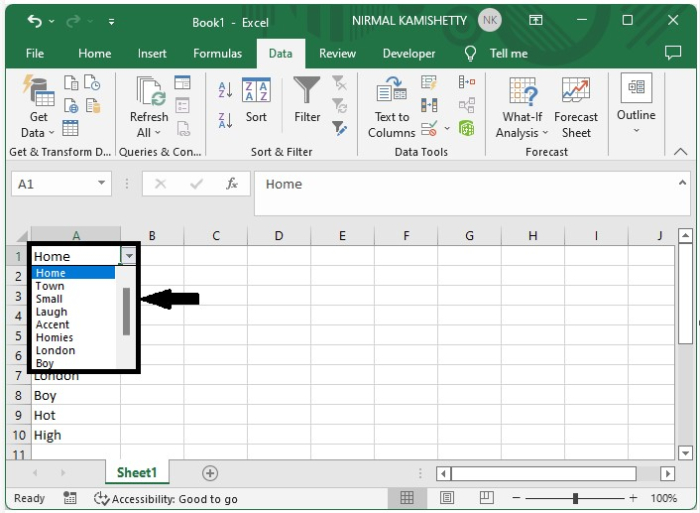Now draw a combo box on the sheet; we can find it under the active X command under developer, as shown in the below image.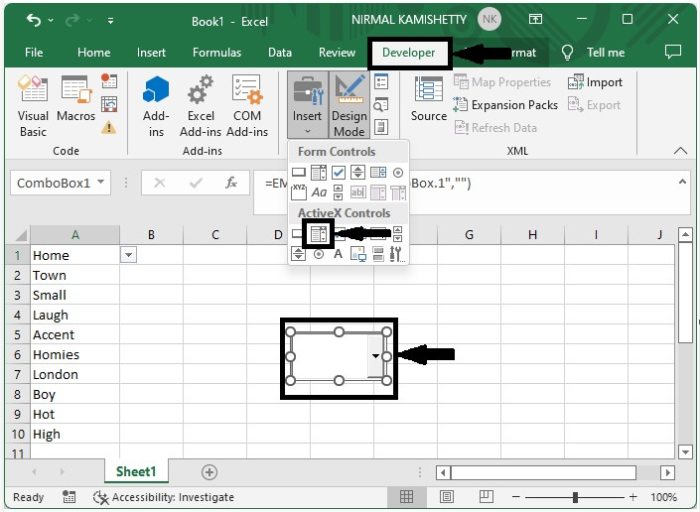Step 2

Now right-click on the box, select properties, and change the name to TempCombo as shown in the below image.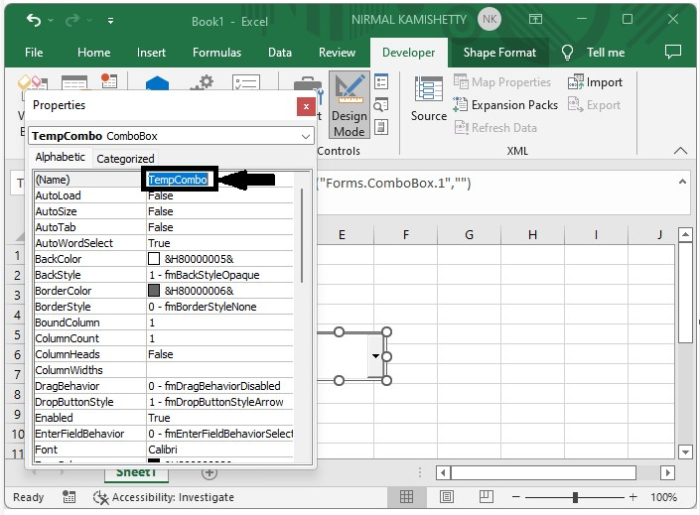Step 3

Then close the properties pop-up and right-click on the sheet name, then select view code to open the VB application, then type the programme into the text box as shown in the below image.

### Program

Private Sub Worksheet_SelectionChange(ByVal Target As Range)
'Update by Nirmal
Dim xCombox As OLEObject
Dim xStr As String
Dim xWs As Worksheet
Dim xArr

Set xWs = Application.ActiveSheet
On Error Resume Next
Set xCombox = xWs.OLEObjects("TempCombo")
With xCombox
.ListFillRange = ""
.Visible = False
End With
If Target.Validation.Type = 3 Then
Target.Validation.InCellDropdown = False
Cancel = True
xStr = Target.Validation.Formula1
xStr = Right(xStr, Len(xStr) - 1)
If xStr = "" Then Exit Sub
With xCombox
.Visible = True
.Left = Target.Left
.Top = Target.Top
.Width = Target.Width + 5
.Height = Target.Height + 5
.ListFillRange = xStr
If .ListFillRange = "" Then
xArr = Split(xStr, ",")
Me.TempCombo.List = xArr
End If
End With
xCombox.Activate
Me.TempCombo.DropDown
End If
End Sub
Private Sub TempCombo_KeyDown(ByVal KeyCode As MSForms.ReturnInteger, ByVal Shift As Integer)
Select Case KeyCode
Case 9
Application.ActiveCell.Offset(0, 1).Activate
Case 13
Application.ActiveCell.Offset(1, 0).Activate
End Select
End Sub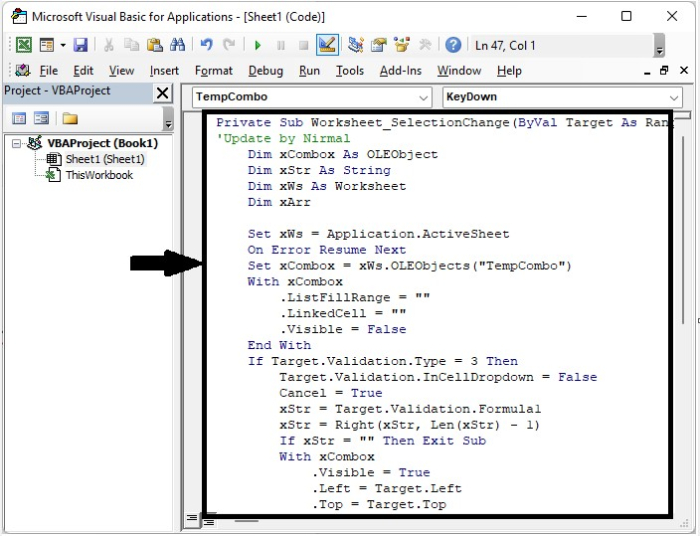Now save the sheet as a macro-enabled template, close the vba application using the command "Alt + Q", then go to the sheet and exit design mode by clicking the design, and our final output will be similar to that shown in the below image.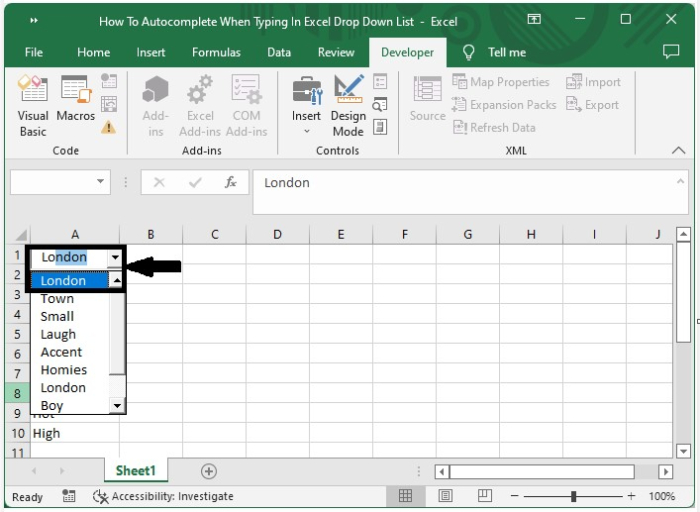## Conclusion

In this tutorial, we used a simple example to demonstrate how you can autocomplete when typing in a dropdown list in Excel.

Updated on: 03-Jan-2023

6K+ Views